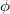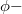﻿

### Lacunary Statistically$\phi$- Convergence

#### Abstract

In this paper by using the lacunary sequence and Orlicz function$\phi$, we introduce a new concept of lacunary statistically$\phi$-convergence, as a generalization of the statistically$\phi-$convergence and$\phi-$convergence. Based on this concepts, introduce a new sequence space$% S_{\theta}-\phi$ and investigate some of its basic properties. Also studied some inclusion relations.

DOI Code: 10.1285/i15900932v39n2p111

Keywords: Orlicz function; lacunary sequence; statistical convergence; $\phi-$convergence

Full Text: PDF